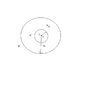# Multiple Choice ?

srhly
Can anybody help me on this question, I'm stuck. I have a picture attached for clarification.

Consider two concentric spherical conducting shells. O is at the center of the shells. THe inner shell has radius a and charge q1 on it, while the outer shell has radius b=3a and charge q2 on it. Find the electric potential at point p, where p is in between the two shells and OP= 2a.

Which represents the electric potential V at point p?
1. V = 0
2. V = infinity
4. V = K[(q1/2a)+(q2/3a)]
5. V = K(q1+q2)/3a
6. V = K(q1/a + q2/3a)

I have already found that answer 3 was wrong so I didn't include it.

#### Attachments

•physicshelp.JPG
8.3 KB · Views: 557